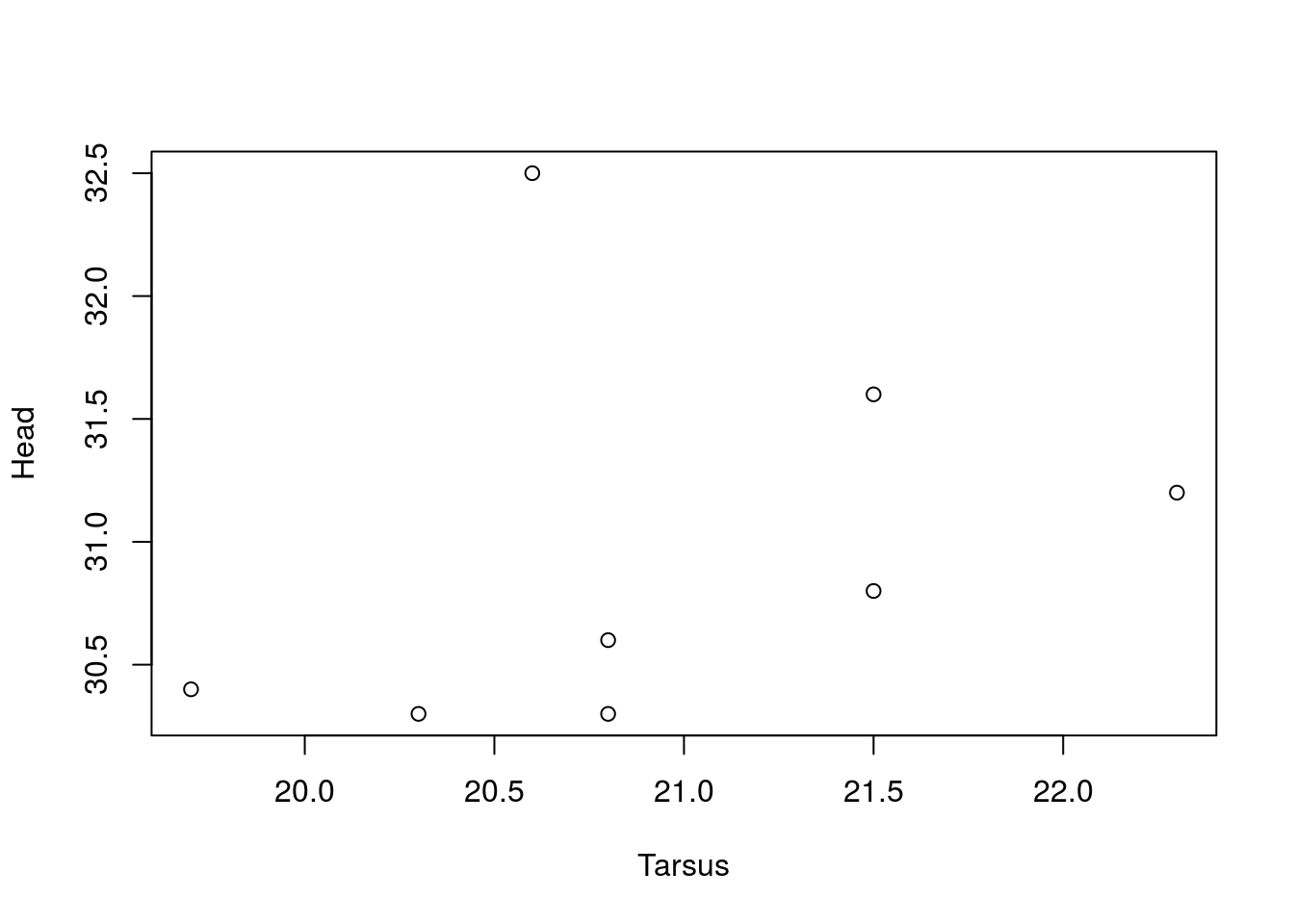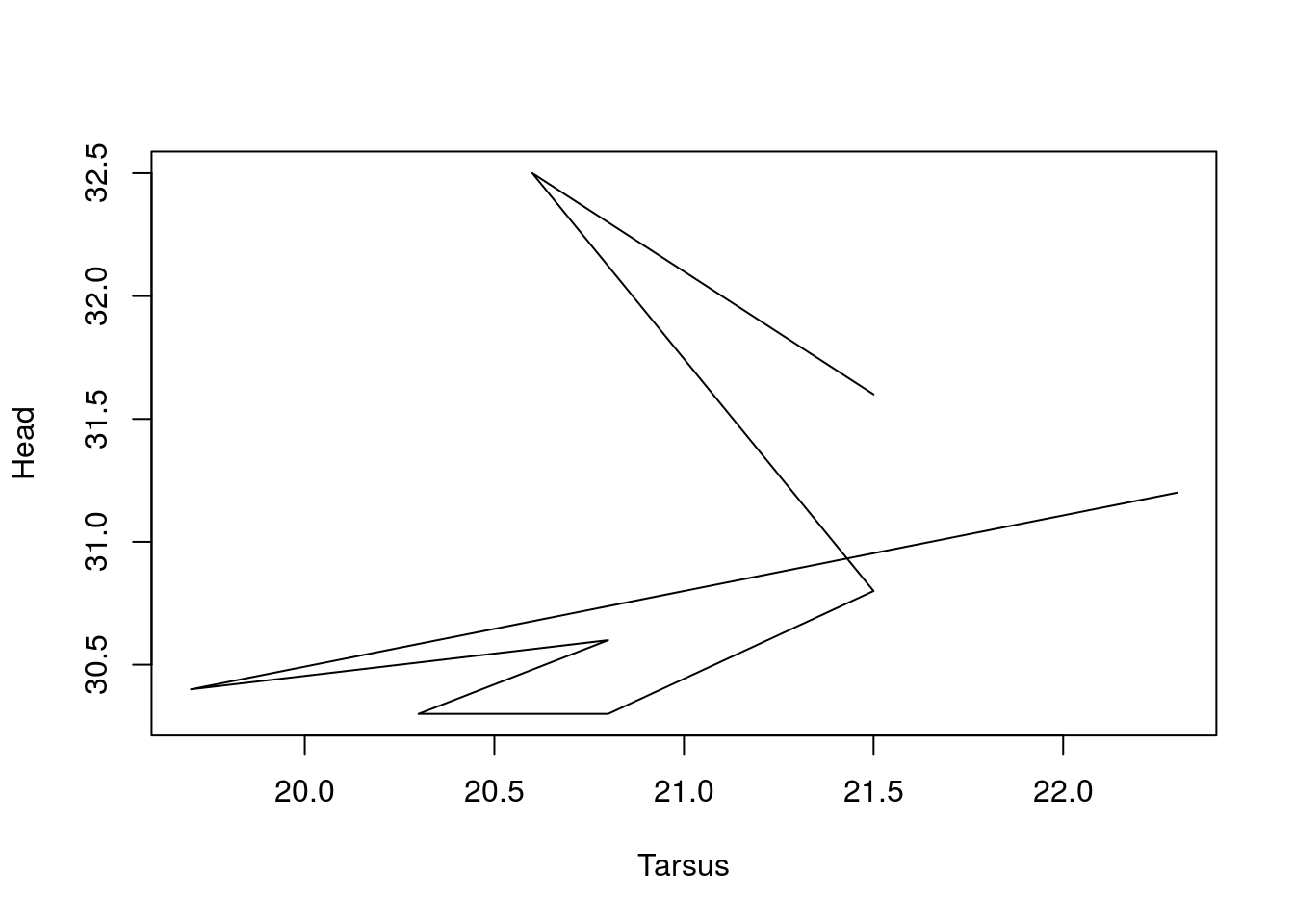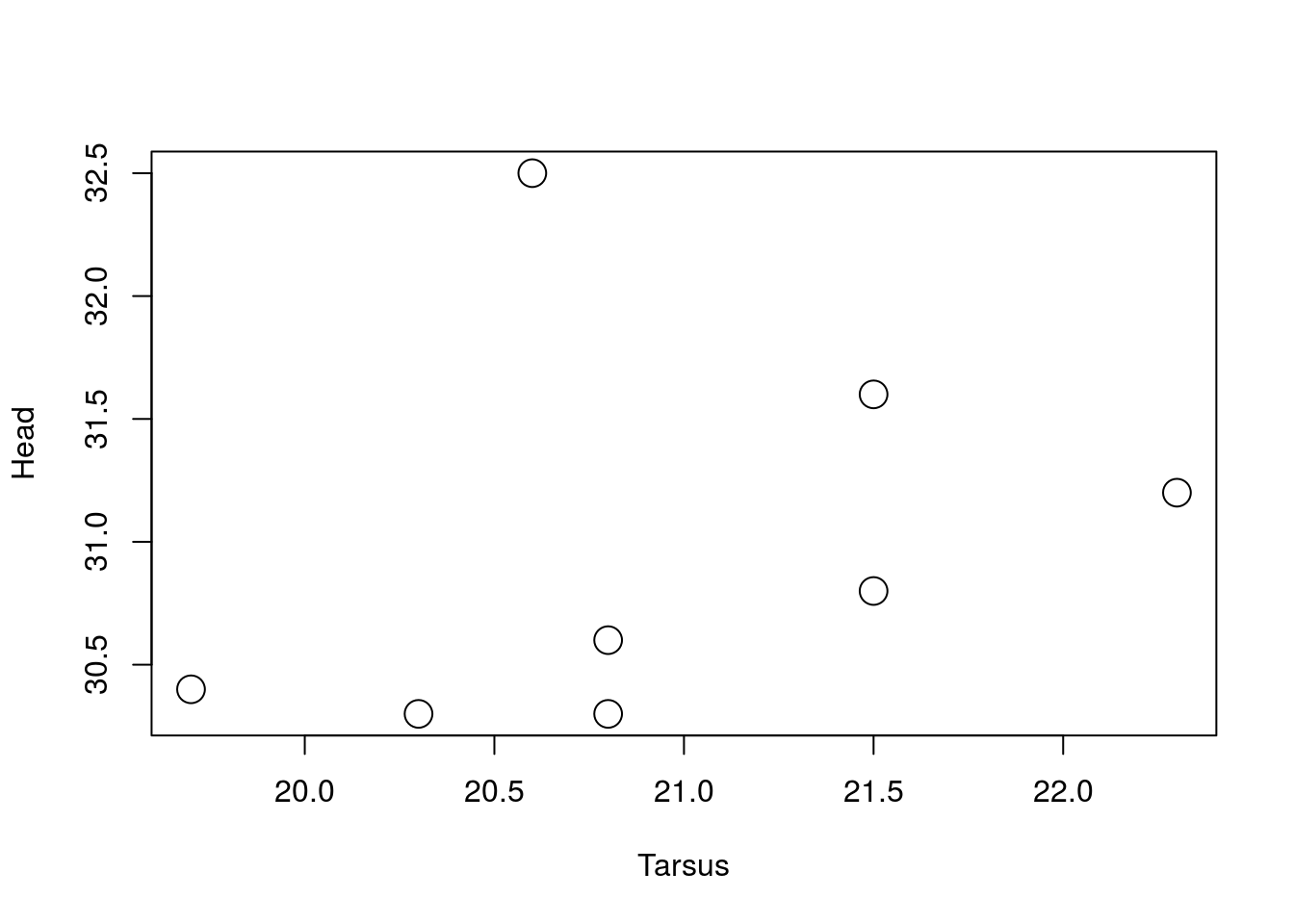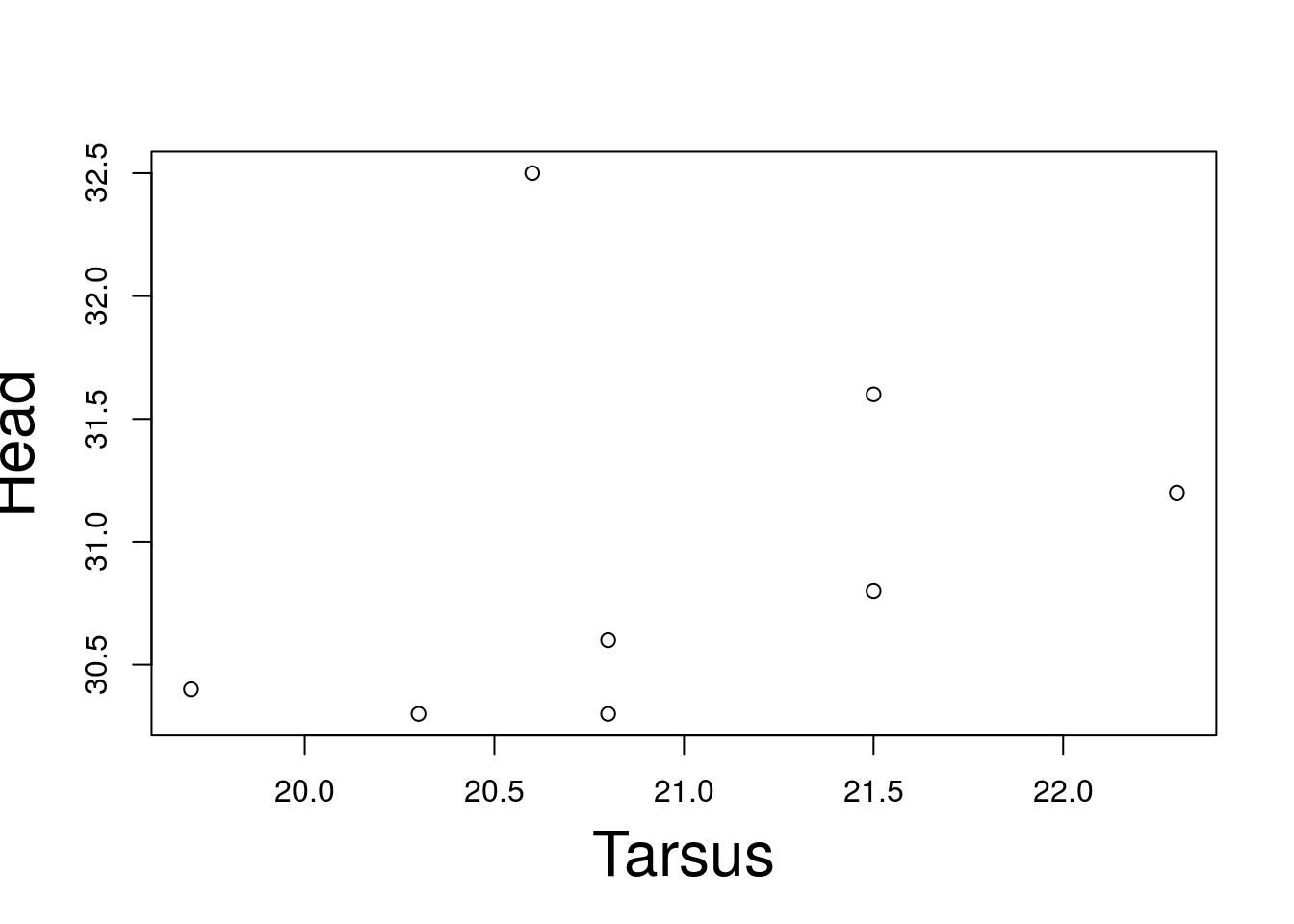Now that you’re equipped to select the perfect plot for your data, let’s learn how to further modify standard plots for our needs.

We will use a small subset of the sparrows dataset, called ‘BirdData’. Look at the data now, type BirdData.

``BirdData``
``````##   Tarsus Head Weight Wingcrd Species
## 1   22.3 31.2    9.5    59.0       A
## 2   19.7 30.4   13.8    55.0       A
## 3   20.8 30.6   14.8    53.5       A
## 4   20.3 30.3   15.2    55.0       A
## 5   20.8 30.3   15.5    52.5       A
## 6   21.5 30.8   15.6    57.5       B
## 7   20.6 32.5   15.6    53.0       B
## 8   21.5 31.6   15.7    55.0       B``````

## Point v line plot

Let’s learn how to set the `type =` argument of our plots, allowing us adjust the exact representation of our data.

Plot Head as a function of Tarsus, setting the data = argument.

``plot(Head ~ Tarsus, data = BirdData)``Now let’s change the `type =` argument to represent the points as a line. Go ahead and re-plot the data, setting the type argument to ‘l’, meaning that you wish to join the points with lines.

``plot(Head ~ Tarsus, data = BirdData, type = 'l')``Not actually a great way to represent our data! But it’s good to know how to do. We often want to convert a scatterplot to a line when we’re plotting longitudinal data, like a time series, where we are interested in the trajectory of a variable. Take a look at the `type =` argument in the `plot()` help file to see other options.

## Changing the size of plot elements

Now lets look at the ‘cex’ (character expansion) argument. We can use ‘cex’ to scale all or some elements in our plot.

By default, `cex =` scales the size of the points in a plot. Go ahead and re-plot `\$Head` as a function of `\$Tarsus`, setting the data argument and `cex = 2`. Revert back to making the default scatterplot.

``plot(Head ~ Tarsus, data = BirdData, cex = 2)```cex =` can also be targeted at specific plotting elements. Plot the same data, but instead of setting `cex =`, set `cex.lab =` to 2.

``plot(Head ~ Tarsus, data = BirdData, cex.lab = 2)``Check the help file for `par()` to see other options for `cex =`.

## A brief digression on colour

While we’ve used colour before, let’s discuss it a little. Colour is very useful for various things in graphics, mostly in terms of illustrating different groups of data. In the past, most journals were only printed in black and white, so different shades of grey were important.

R has 102 unique shades of grey. See this blogplost, if you want: https://www.r-bloggers.com/50-shades-of-grey-according-to-r/.

R also has access to a very wide range of colours (including grey). Colours can be accessed in a variety of ways; (i) by number, e.g., `col = 1` for black, or `col = 2` for red; (ii) by name, e.g., `col = 'black'`; (iii) by hexadecimal code, e.g., `col = '#FF0000'`; (iv) or RGB value, e.g., `col = rgb(0,0,0)`. We can even give colors transparency with `rgb()` using the ‘alpha’ argument.

Try changing the colour of the data points in the figure to red, using the name. The colours will be more obvious if you also use a different plotting character, so use `pch = 20`.

``plot(Head ~ Tarsus, data = BirdData, pch = 20, col = 'red')``